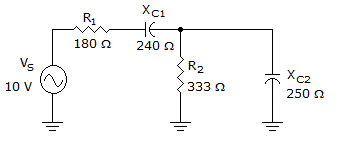# Electronics - RC Circuits

Exercise : RC Circuits - General Questions
1.
In a 20 Vac series RC circuit, if 20 V is read across the resistor and 40 V is measured across the capacitor, the applied voltage is
45 Vac
50 Vac
60 Vac
65 Vac
Explanation:
No answer description is available. Let's discuss.

2.
Which of the following is the reference vector for parallel RC circuits?
R
V
I
XC
Explanation:
No answer description is available. Let's discuss.

3.What is the voltage drop across R1 in the given circuit?

10 V
4.80 V
4.00 V
5.80 V
Explanation:
No answer description is available. Let's discuss.

4.
Power that is measured in volt-amperes is called
impedance power
reactive power
true power
apparent power